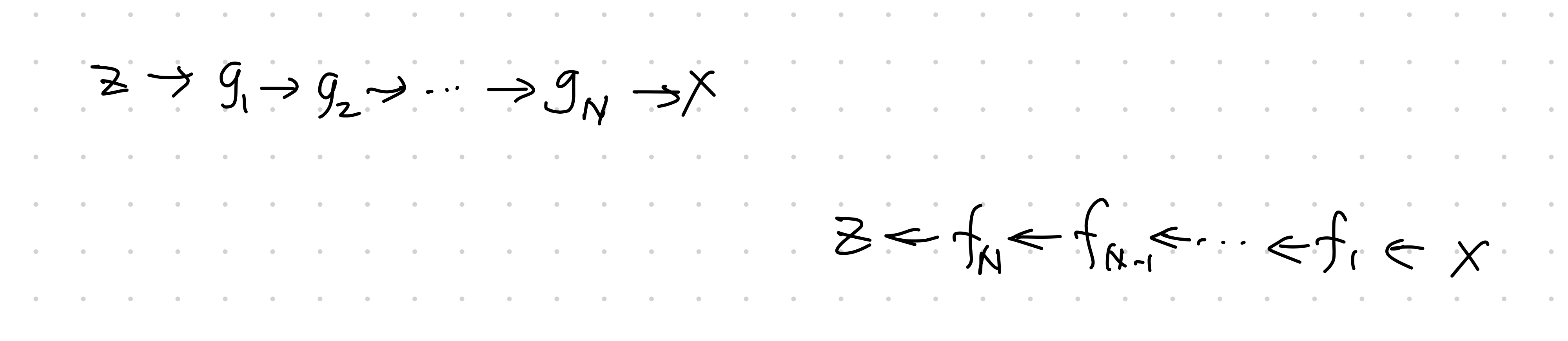# Flow¶For a probability density $$p(x)$$ and a transformation of coordinate $$x=g(z)$$ or $$z=f(x)$$, the density can be expressed using the coordinate transformations, i.e.,

\begin{align} p(x) &= \tilde p (f(x)) \lvert \operatorname{det} \operatorname{D} g(f(x)) \rvert^{-1} \\ &= \tilde p(f(x)) \lvert \operatorname{det}\operatorname{D} f(x) \rvert \end{align}

where the Jacobian is

$\operatorname{D} g(z) \to \frac{\partial }{\partial z} g.$

The operation $$g_{*}\circ \tilde p(z)$$ is the push forward of $$\tilde p(z)$$. The operation $$g_{*}$$ will pushforward simple distribution $$\tilde p(z)$$ to a more complex distribution $$p(x)$$.

• The generative direction: sample $$z$$ from distribution $$\tilde p(z)$$, apply transformation $$g(z)$$;
• The normalizing direction: "simplify" $$p(x)$$ to some simple distribution $$\tilde p(z)$$.

The key to the flow model is the chaining of the transformations

$\operatorname{det} \operatorname{D} f(x) = \Pi_{i=1}^N \operatorname{det} \operatorname{D} f_i (x_i)$

where

\begin{align} x_i &= g_i \circ \cdots \circ g_1 (z)\\ &= f_{i+1} \circ \cdots \circ f_N (x). \end{align}

1. Liu X, Zhang F, Hou Z, Wang Z, Mian L, Zhang J, et al. Self-supervised Learning: Generative or Contrastive. arXiv [cs.LG]. 2020. Available: http://arxiv.org/abs/2006.08218

Contributors: LM